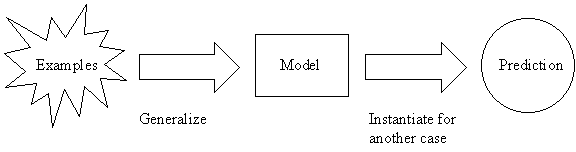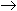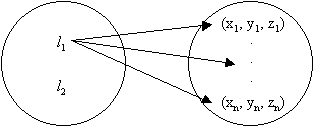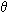Inductive inference is the process of reaching a general conclusion from specific examples.The general conclusion should apply to unseen examples.

Inductive Learning Hypothesis: any hypothesis found to approximate the target function well over a sufficiently large set of training examples will also approximate the target function well over other unobserved examples.

Example:

Identified relevant attributes: x, y, z

 x y z 2 3 5 4 6 10 5 2 7

Model 1:

x + y = z

Prediction: x = 0, z = 0y = 0

Model 2:

if x = 2 and z = 5, then y = 3.
if x = 4 and z = 10, then y = 6.
if x = 5 and z = 7, then y = 2.
otherwise y = 1.

Model 2 is likely overfitting.

Good:
completely consistent with data.
no justification in the data for the prediction that y = 1 in all other cases.
not in the class of algebraic functions (but nothing was said about class of descriptions).

Inductive bias: explicit or implicit assumption(s) about what kind of model is wanted.

Typical inductive bias:

• prefer models that can be written in a concise way.
• Select the shortest one.

Example:

• The decision tree ID3 algorithm searches the complete hypothesis space, and there is no restriction on the number of hypthotheses that could eventually be enumerated. However, this algorithm searches incompletely through the set of possibly hypotheses and preferentially selects those hypotheses that lead to a smaller decision tree. This type of bias is called a preference (or search) bias.
• In contrast, the version space candidate-elimination algorithm searches through only a subset of the possible hypotheses (an incomplete hypothesis space), yet searches this space completely. This type of bias is called a restriction (or language) bias, because the number of possible hyptheses considered is restricted.
• A preference bias is generally more desirable than a restriction bias, because an algorithm with this bias is allowed to search through the complete hypothesis space, which is guaranteed to contain the target function.
• Restricting the hypothesis space being searched (a restriction bias) is less desirable because the target function may not be within the set of hypotheses considered.

Some languages of interest:

• Conjunctive normal form for Boolean variables A, B, C.
• e.g., ABC
• Disjunctive normal form.
• e.g., A(~B)(~C) + A(~B)C + AB(~C)
• Algebraic expressions.

Positive and Negative Examples

Positive Examples

• Are all true.
 x y z 2 3 5 2 5 7 4 6 10

 general x, y, zI more specific x, y, zI+ more specific than the first two 1 < x, y, z < 11 ; x, y, zI even more specific model x + y = z

Negative Examples

• Constrain the set of models consistent with the examples.
 x y z Decision 2 3 5 Y 2 5 7 Y 4 6 10 Y 2 2 5 N

Search for Description

Description keeps getting larger or longer.

Finite language - algorithm terminates.

Infinite language - algorithm runs

• Forever.
• Until out of memory.
• Until a final answer is reached.

X = example space/instance space (all possible examples)

D = description space (set of descriptions defined as a language L)

• Each description lL corresponds to a set of examples Xl.Success Criterion

Look for a description lL such that l is consistent with all observed examples.

• description can be relaxed to match all instances.
• inductive bias can be expressed in the success criterion.

Example:

L = {x op y = z}, op = {+, -, *, /}

Given a precise specification of language and data, write a program to test descriptions one by one against the examples.

• finite language: size = |L|
• finite number of examples: size = |X|
•(|L| * |X|)

Why is Machine Learning Hard (Slow)?

It is very difficult to specify a small finite language that contains a description of the examples.

e.g., algebraic expressions on 3 variables is an infinite language